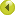Tensors, Relativity and Cosmology Eric A Lord Synopsis Tensors, Relativity and Cosmology provides the student of mathematics or physics with a thorough introduction to the Special and the General theories of Einstein. The first section of the book prepares the mathematical foundations, explaining the algebraic and differential properties of tensors, and the geometrical significance of the metric, the affine connection and the curvature tensors. The section on Special Relativity includes a discussion of the structure of the Lorentz group and its representations, leading to the concept of spinors and relativistic wave equations. The General Theory is introduced by showing why curved spacetime becomes necessary to achieve a relativistic formulation of the equivalence principle and incorporate gravitation. Finally, various historic attempts to extend General Relativity are explained, including Weyl's "gauge" theory, the Unified Theory of Einstein & Schroedinger, Kaluza's five-dimensional formulation, Brans-Dicke theory and Kibble-Sciama theory.